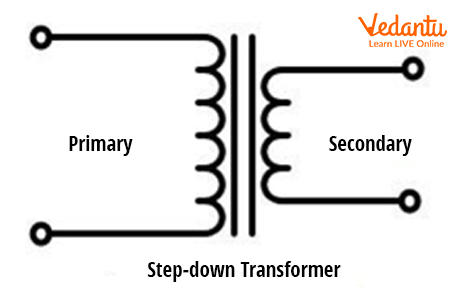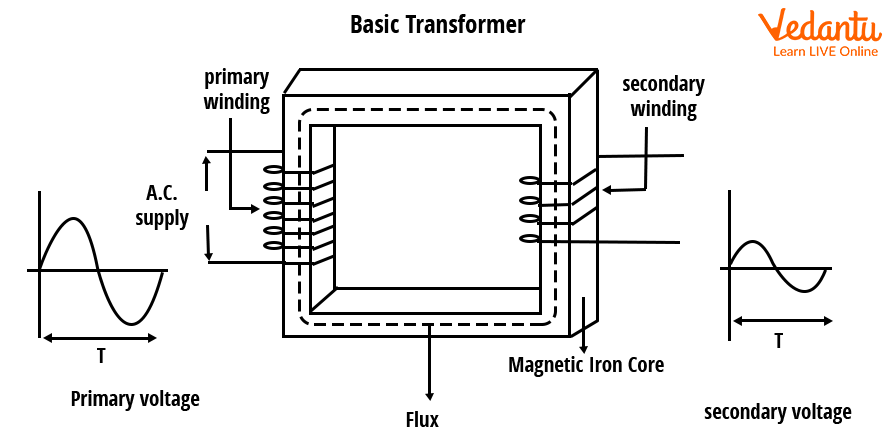# Step Down Transformer Construction and Working for JEE## General Idea about Step Down Transformer

Transformers are stationary electrical devices that transform electrical power between voltage and current settings. Depending on their purpose, transformers are classified as step up or step down transformers. The incoming current voltage is reduced by down transformers while step-up transformers increase incoming current voltage. The secondary voltage is the voltage that goes out whereas the primary voltage is the one that comes in. With no moving parts, a step-down transformer functions as a fixed device transporting electrical power from one circuit to another. During this process, there is no change in frequency, but there is a change in voltage and current.

Step-down transformers are used in high-voltage electrical distribution networks to ensure minimum loss in the electrical sector. It is a cost-effective long-distance power supply option. To convert a high voltage supply line to a low voltage line, a step-down transformer is utilized. In both the electronic and electrical sectors, step-down transformers are used. A step-down transformer reduces the primary voltage level to a lower level via the secondary output. To do this, the main and secondary winding ratios are used. In step-down transformers, the primary side has more windings than the secondary side.

## What is a Step Down Transformer?

In both the electronic and electrical sectors, step-down transformers are used. A step-down transformer reduces the primary voltage level to a lower level via the secondary output. To do this, the main and secondary winding ratios are used. In step-down transformers, the primary side has more windings than the secondary side.

## Construction of Step Down Transformer

Steel bands surround the iron core of a step-down transformer. Lamination insulated thin metal strips make up the majority of the seats. Aside from that, the primary and secondary winding types have two sides. Both windings are independent of each other and are created by the electric coil, as stated in the following sections.

### 1. Winding

Wires with low resistance and good conductivity are used in step down transformers, which aid in the transformer's efficiency. Copper is used in the winding of step-down transformers. Wires are called coils to rotate the transformer at the same time.Step down transformer symbol

### 2. Core

Ferromagnetic materials with high permeability are used to make the cores. This is done with iron. The cores of step-down transformers are built of a highly absorbent substance capable of transporting current. A step-down transformer's cores act as a route for magnetic flux to move smoothly. The core's primary function is to make magnetic flux winding easier while supplying a good change in a less cost-effective direction.

## Step Down Transformer Working Principle

A step-down transformer operates primarily on the basis of electromagnetic induction. A conductor in a fluctuating electromagnetic field will see an induced current dependent on the rate at which the flux changes, according to Faraday's first law of electromagnetic induction. The main and secondary windings of the transformer have a high mutual induction and a common magnetic field because they are made up of two coils. As a result, the primary winding can cause a current in the secondary.

## Working on Step Down Transformer

An emf in the second coil, coupled to the first coil, is generated by a uniform current variation in one coil. Mutual inductance is also used in the operation of an electric transformer. Two coils with a large mutual inductance are used in a step-down transformer, and these two coils are electrically isolated. In a step-down transformer, the primary or primary winding coil is the initial set of coils connected to a direct voltage and an alternating voltage source on the first side. The load is connected to the second set of coils, the secondary winding or secondary coil. This load draws the alternating result voltage. The windings of a step-down transformer carry alternating electricity.

The primary winding is excited by the alternating input voltage. This alternating current generates an alternating magnetic flux that travels through the magnetic iron core and back.Step down transformer circuit diagram

Because the secondary winding is likewise connected to an alternating magnetic flux, an E.M.F is formed in the secondary winding, according to Faraday's law. The number of windings is determined by the voltage intensity traveling through the secondary winding, where the primary winding alternating voltage is passed to the other winding. Compared to the transformer's primary side, the voltage on the secondary side is the same. The voltage's frequency and duration remain constant.

## Step Down Transformer Formula

The voltage-to-voltage ratio is determined by the turn ratio of a step-down transformer. If the transformer's output voltage is higher, it signifies the transformer's windings have a more significant number of turns. The AC supply voltage levels are higher in step-down transformers. To counteract, the primary has more spins while the secondary has less power, resulting in lower voltage.

Turns ratio = $\frac{V_{p}}{V_{s}}$ = $\frac{N_{p}}{N_{s}}$

where

• Vs = Secondary coil voltage

• Vp = Primary coil voltage

• Np = The number of turns in the primary coil

• Ns = Number of secondary coil turns

## Use of Step Down Transformer

Step down transformers have a wide range of uses, however, the following are the most common.

• In electronic SMPS, step-down transformers are employed. Step down transformers are also used in rectifiers and power adapters to reduce voltage efficiently.

• Power transmission lines, welding machines, and inverters all employ step-down transformers.

• Voltage stabilizers and inverters are examples of step down transformers.

## Conclusion

High currents and low voltages can be provided via step-down transformers. Step-down transformers have the advantage of being able to provide the appropriate output with a 99 percent efficiency. As a result, they are less costly and more dependable. Step-down transformers are highly efficient, allowing us to attain the desired output voltage with minimal power loss.

## FAQs on Step Down Transformer Construction and Working for JEE

1. What is a step down transformer and how does it step down voltage?

Faraday's law of electromagnetic induction is the working principle of a step-down transformer in most cases. Mutual induction is required for transmission in a transformer between two windings. A step-down transformer is actually fairly basic in concept. On the primary coil of the transfer, there are more wire turns than on the secondary coil. The induced voltage going through the secondary coil is reduced as a result, and the output voltage is reduced as a result. Stabilizers used as voltage step down converters are common examples of step down transformers.

2. Which of a step down transformer's windings will carry the most current?

The step-down transformer's secondary voltage is lower than its primary voltage. The primary winding should have more turns as compared to the secondary winding. The primary winding has a higher number of turns than the secondary winding. The transformer's primary current is greater than the secondary current. This low voltage value, which is ideal for electronics supply, is provided by a step-down transformer.

3. What role does a step-down transformer play?

A step-down transformer works in the same way as a traditional transformer. A step-down transformer uses Faraday's law and the Turn Ratio to produce low output voltage is produced from the high input voltage. Current travels across the wires as a result of the applied voltage in the primary windings. In a power system, step-down transformers play a crucial role. They reduce the voltage and tailor it to the needs of energy customers. Because the secondary winding has fewer turns than the primary winding, the secondary voltage in a step-down transformer is lower than the primary voltage. As a result, this transformer is utilized to reduce the voltage to the circuit's intended levels.

Comment